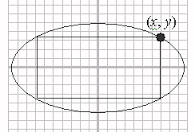Question -19

Calculus Level 3Area of the greatest rectangle that can be inscribed in the ellipse $\dfrac{x^2}{a^2}+\dfrac{y^2}{b^2}=1$ is :

×